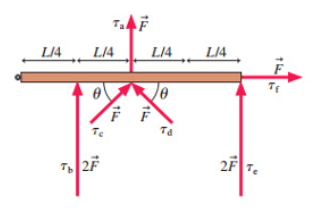# Problem: Six forces are applied to the door in (Figure 1).Rank in order, from largest to smallest, the six torques τa to τf about the hinge on the left.

###### FREE Expert Solution

Torque:

$\overline{){\mathbf{\tau }}{\mathbf{=}}{\mathbf{r}}{\mathbf{F}}{\mathbf{s}}{\mathbf{i}}{\mathbf{n}}{\mathbf{\theta }}}$

τa = (L/2)(F)•sin90 = LF/2

τb = (L/4)2F•sin90 = LF/2

94% (404 ratings)###### Problem Details

Six forces are applied to the door in (Figure 1).Rank in order, from largest to smallest, the six torques τa to τf about the hinge on the left.